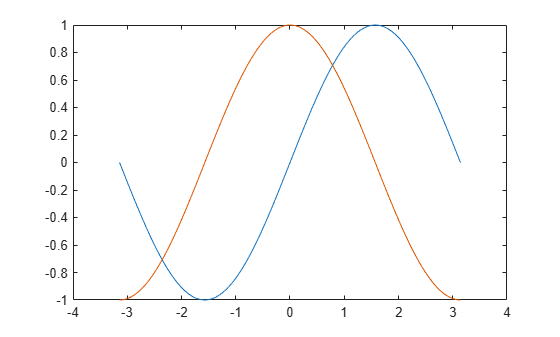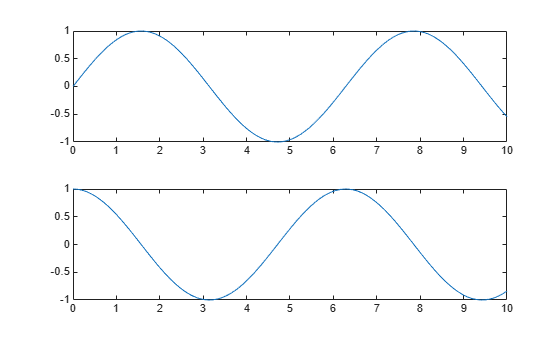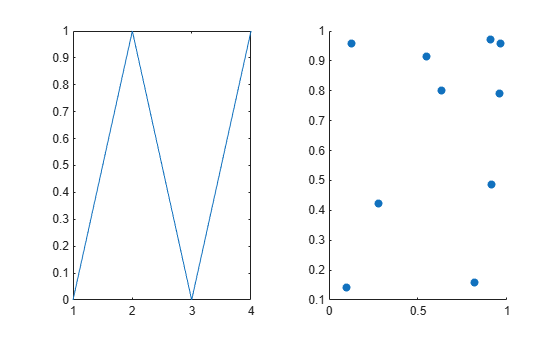# hold

## 语法

``hold on``
``hold off``
``hold all``
``hold``
``hold(ax,___)``

## 说明

``hold on` 保留当前坐标区中的绘图，从而使新添加到坐标区中的绘图不会删除现有绘图。新绘图基于坐标区的 `ColorOrder` 和 `LineStyleOrder` 属性使用后续的颜色和线型。MATLAB® 将调整坐标区的范围、刻度线和刻度标签以显示完整范围的数据。如果不存在坐标区，`hold` 命令会创建坐标区。`

``hold off` 将保留状态设置为 off，从而使新添加到坐标区中的绘图清除现有绘图并重置所有的坐标区属性。添加到坐标区的下一个绘图基于坐标区的 `ColorOrder` 和 `LineStyleOrder` 属性使用第一个颜色和线型。此选项为默认行为。`
``hold all` 与 `hold on` 相同。在以后的版本中将会删除该语法。请改用 `hold on`。`
``hold` 在 on 和 off 之间切换保留状态。`

``hold(ax,___)` 为 `ax` 指定的坐标区而非当前坐标区设置 hold 状态。请在前面任何语法中的所有其他参数之前指定 `ax`。使用单引号将 `'on'` 和 `'off'` 输入引起来，例如 `hold(ax,'on')`。`

## 示例

```x = linspace(-pi,pi); y1 = sin(x); plot(x,y1) hold on y2 = cos(x); plot(x,y2) hold off``````y3 = sin(2*x); plot(x,y3)``````x = linspace(0,10); y1 = sin(x); y2 = cos(x); tiledlayout(2,1) % Top plot ax1 = nexttile; plot(ax1,x,y1) % Bottom plot ax2 = nexttile; plot(ax2,x,y2)``````hold(ax1,'on') y3 = sin(2*x); plot(ax1,x,y3) hold(ax1,'off')``````t = tiledlayout(1,2); ax1 = nexttile; ax2 = nexttile; plot(ax1,[0 1 0 1]) scatter(ax2,rand(1,10),rand(1,10),'filled')``````hold([ax1 ax2],'on') plot(ax1,[.5 .2 .5 .2]) scatter(ax2,rand(1,10),rand(1,10),'filled')```## 输入参数

• 任何类型的坐标区对象：`Axes``PolarAxes``GeographicAxes` 对象。

• 属于同一类的坐标区对象组成的数组。要确定类，请使用 `class` 函数。

## 提示

• 使用 `ishold` 函数测试保留状态。

## 算法

`hold` 函数设置以下属性：

• `NextPlot` 坐标区属性 - 将相关联的 `Axes``PolarAxes``GeographicAxes` 对象的此属性设置为 `'add'``'replace'`

• `NextPlot` 图窗属性 - 将 `Figure` 对象的此属性设置为 `'add'`